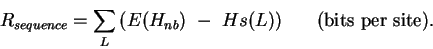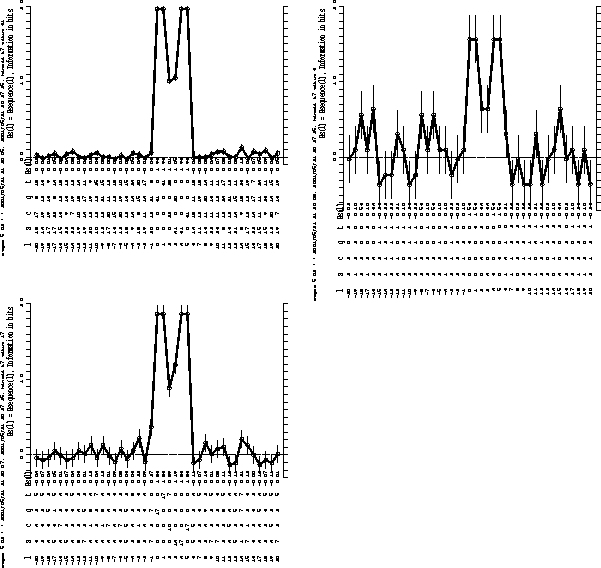>Next: (iii) Determining the Binding Up: (a) Calculation of Rsequence Previous: (i) Formula for Rsequence

### (ii) Graphs of Rsequence and Correction for Sampling Error

In Fig. 1, we show the curve Rsequence(L) for either 61 (a), 17 (b) or 6 (c) HincII sites (GTPyPuAC; Roberts, 1983) chosen from the left end of bacteriophage T7 (Dunn and Studier, 1983). Here, the G's in the HincII sites have been placed at position L=0, and Rsequence(L) was calculated for 20 bases on either side. There are two major 2-bit peaks of information content surrounding a 1-bit valley in curve (a). None of the curves go to zero (the solid straight line) outside the sites, although they come close at several points. This effect is not small: for six sites (Fig. 1c) the background is at 0.44 bits per base so that with sequences 41 bases long, Rsequence will be overestimated by 18 bits. A sampling error correction for Hs(L)(e(n), Appendix I, page). can be joined with Hg to give the final formula:(6)

With this correction, the information content measured at various positions of an aligned set of random sequences will vary above and below zero. On the average it should be zero outside a binding site. The information content inside a site will rise above zero. These features can be seen in all figures, where the corrected zero is shown as a dashed line.The numbers of bases at each position, n(B,L), are given. The sites were obtained starting at the left end of the bacteriophage T7 DNA sequence (Dunn and Studier, 1983) and only one orientation of each site was used. The left-most base in each site (G) was placed at position 0 in each case, and the sequence examined for 20 nucleotides in each direction from this base. The solid lines are the zero without sampling error correction. The dashed lines are the zero when the correction is made. The bars show one standard deviation above or below Rsequence(L). They show the variation of the sampling error correction. (a) 61 sites, Rsequence = 10.70.2 bits; (b) 17 sites, Rsequence = 9.90.7 bits; (c) 6 sites, Rsequence = 8.32.0 bits.

The standard deviation reported for each Rsequence is based on the variance of Hnb (Appendix I, page) which is sensitive to the number of sequence examples, but not to the actual sequences. It is only a measure of variance in the correction for small sample sizes; the variation in the information content of individual sites will be described elsewhere. The variance of the sampling correction is shown in all figures as a bar extending one standard deviation above and below the Rsequence(L) curve.Next: (iii) Determining the Binding Up: (a) Calculation of Rsequence Previous: (i) Formula for Rsequence
Tom Schneider
2002-10-16